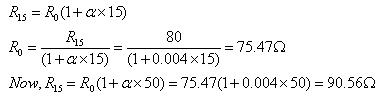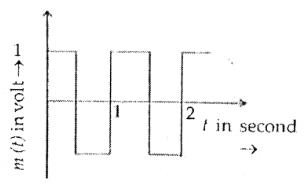Courses

# Test: Drift Of Electrons And Resistivity

## 20 Questions MCQ Test Physics Class 12 | Test: Drift Of Electrons And Resistivity

Description
This mock test of Test: Drift Of Electrons And Resistivity for Class 12 helps you for every Class 12 entrance exam. This contains 20 Multiple Choice Questions for Class 12 Test: Drift Of Electrons And Resistivity (mcq) to study with solutions a complete question bank. The solved questions answers in this Test: Drift Of Electrons And Resistivity quiz give you a good mix of easy questions and tough questions. Class 12 students definitely take this Test: Drift Of Electrons And Resistivity exercise for a better result in the exam. You can find other Test: Drift Of Electrons And Resistivity extra questions, long questions & short questions for Class 12 on EduRev as well by searching above.
QUESTION: 1

### Conversion of temperature into electric voltage is done with

Solution:

To convert temperature to voltage we can do a precise measurement of the temperature in a room. A NTC resistor or a thermistor
It is used as a sensor that has a strong temperature dependence.

QUESTION: 2

### For semiconductors, the major factor affecting the value of resistivity when temperature changes is

Solution:

n increases with temperature and this increase more than compensates any decrease in relaxation time. Hence, for semiconductors resistivity decreases with the increase in temperature.

QUESTION: 3

### When a conductor is placed in an external electric field, the acceleration of its electrons will be

Solution:

F=-eE .
we know that,
F= ma ,
then
a=F/m.
a=-eE/m.

QUESTION: 4

Resistors can be wire bound or carbon resistors. Wire bound resistors are generally made of

Solution:

A Nichrome or manganin is commonly used as the metal wires in wire-wound resistors because they provide high resistance to the electric current and operate at high temperature.

QUESTION: 5

The dimension of the temperature coefficient of resistivity is​

Solution:

The resistance of a material at temperature T is given by
R=R0​[1+α(T−T0​)]
Where R0​= resistance at reference temperature T​0=0oC and α is the temperature coefficient of resistance.
From the above equation, the unit of the temperature coefficient is per degree Celsius (/oC). So, dimension will be [C−1]

QUESTION: 6

The winding of a motor has a resistance of 80 ohms at 15°C. Find its resistance at 50° C. the temperature co-efficient of resistance is 0.004/°C​

Solution:QUESTION: 7

On heating a conductor its resistance

Solution:

Heating a conductor makes it more difficult for electricity to flow through it. These collisions cause resistance and generate heat.Heating the conductor causes atoms to vibrate more, which in turn makes it more difficult for the electrons to flow, increasing resistance.

QUESTION: 8

Specific resistance of a conductor increases with

Solution:

Resistance of a conductor is given by R = ρ l/A,
where ρ is the specific resistance, l is the length and A is the cross-sectional area of the conductor.
Now, when l = 1 and A = 1, R = ρ. So specific resistance or resistivity of a material may be defined as the resistance of a specimen of the material having unit length and unit cross-section. Hence, specific resistance is a property of a material and it will increase with the increase of temperature, but will not vary with the dimensions (length, cross section) of the conductor.

QUESTION: 9

A steady current flows in a metallic conductor of non-uniform cross-section. The quantity/quantities constant along the length of the conductor is/are

Solution:

Current does not depend on the area of the conductor; hence it remains a constant.
Current density is inversely a constant.
Current density is inversely proportional to area (i.e.,) J∝1/A​ electric field drift speed is also inversely proportional to area (i.e.,) E∝1/A​,Vd​∝1/A​
Hence current is constant along the conductor.

QUESTION: 10

A current of 2 ampere is passing through a metallic wire of cross-sectional area 2 x 10-6 m2. If the density of the charge carriers in the wire is 5 x 1026 m-3, then the drift velocity of the electrons will be​

Solution:

Drift velocity, v=I/nAe
=2/(2x10-6)x(5x1026)x(1.6x10-19)
=1.25x10-2m/s

QUESTION: 11

The standard resistance coil are made of “manganin” because

Solution:

Solution :- The temperature co-efficient of resistance of manganin is very low. Hence its resistance is almost independent of temperature. Hence it is used in making standard resistances.

QUESTION: 12

If the potential difference V applied on a conductor is doubled, the drift velocity of electrons will become

Solution:
QUESTION: 13

When a conductor is placed in an electric field, the force experienced by its electrons will be

Solution:
QUESTION: 14

Resistance of a conductor depends on

Solution:

The resistance of a conductor depends on thickness (cross sectional area of the wire), length and temperature.
Resistivity is defined as the measure of the resisting power of a specified material to the flow of an electric current.
R=ρ(I/A)​
where R = Resistance of the conductor
ρ = Resistivity of the conductor
l = length of the conductor
A = Area of cross section
i.e., ρ=R (A​/l)

QUESTION: 15

A carbon resistor is marked in green, red, and orange bands. The approximate resistance of the resistor is

Solution:

the first colour shows the unit digit
the second colour shows the tens digit
the third colour shows the power of 10
the fourth colour shows the tolerance
green=5
red=2
orange=3
no colour=20%
therefore approximate resistance=52x10​3Ω and tolerance of 20%

QUESTION: 16

Two special characteristics of the element of an electric heater:

Solution:

When we use a heating element that has
1. High specific resistance: a small quantity of wire is used to generate the required amount of heat or else the quantity of wire would be required higher in order to heat.
2. High melting point: a higher melting point is selected so that higher temperature is attained. Nickel and chromium alloys are ideal as a heating element.

QUESTION: 17

The type of materials whose resistivity is affected on adding the impurity is known as

Solution:

Semiconductors are materials which have a conductivity between conductors (generally metals) and nonconductors or insulators (such as most ceramics). Semiconductors can be pure elements, such as silicon or germanium, or compounds such as gallium arsenide or cadmium selenide. In a process called doping, small amounts of impurities are added to pure semiconductors causing large changes in the conductivity of the material.

QUESTION: 18

The average time that elapses between two successive collisions of an electron is called

Solution:

Relaxation time is the average time between two successive collisions between the free electrons and the atoms(kernel) of atoms .
It is denoted by τ.

QUESTION: 19

Mobility of charge carriers in a conductor is given by​

Solution:

Mobility of charge carriers in a conductor is defined as the magnitude of their drift velocity per unit applied electric field.
Mobility,
μ=Drift of electric field
μ=VdE
S.I. unit of mobility is m2Vs or ms NC.QUESTION: 20

Manganin and constantan have a low temperature coefficient of resistivity which means that

Solution:

Constantan is an alloy of copper (55%) and nickel (45%) where Manganin is an alloy of copper (84%) manganese(12%) and nickel (4%).
When all metals show increase in resistivity with increase in temperature and semiconductors show the reverse, Constantan and Manganin show a completely different property.
Their main feature is their low thermal variation of their resistivity, which is constant over a wide range of temperatures.
Metals, including alloys, have free electrons as charge carriers. Their movement controls by defects. One of defects of usual metal is oscillations of atoms due to temperature. More temperature - more oscillations - more collisions of electrons with atoms - less mobility - more resistivity. In alloys, like constantan and Manganin, atoms are in disorder. So they have big resistivity. Their additional disorder due to temperature increase is insignificant. That is why they have no temperature dependence on resistivity.